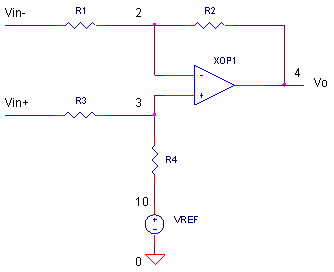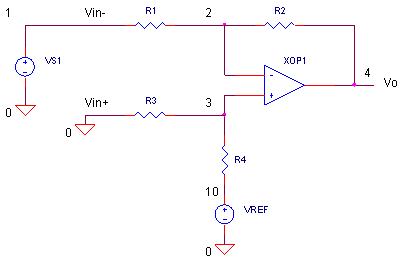eCircuit  Center

### ADC Input Driver SINGLE-ENDED to SINGLE-ENDED

CIRCUITSuppose your sensor produces a bipolar voltage swing of ±10V. The only problem, your ADC accepts a single-ended input of 0 - 5 V. What you need is a circuit that will reduce your voltage swing. Not only that, you'll need to add an offset so your signal is positive only! The handy op amp circuit shown above, takes a single-ended input, then both scales and shifts it to a single-ended signal of your desires.

HOW IT WORKS

First we'll get a handle on how this op amp circuit works, then well apply it to our sensor signal. Let's assume there's one of two possible places you can connect your input: Vin+ or Vin-. We've also got a fixed source to work with, VREF. Now, how do you calculate Vo driven by all three sources? One easy way is by supersposition. Simply calculate Vo due to one source, with the other two sources set to zero. Then repeat for the other sources. Finally, add all of the outputs together.

 Input Conditions Output Vin+ Vin- = VREF = 0V Vo1 = Vin+  ∙  R4/(R3 + R4)  ∙  (R1 + R2)/R1     Assuming R2/R1 = R4/R3 we get Vo1 = Vin+ ∙ (R2/R1) Vin- Vin+ = VREF = 0V Vo2 = Vin- ∙ (- R2/R1) VREF Vin+ = Vin- = 0 V Vo3 = VREF  ∙  R3/(R4 + R3)  ∙  (R1 + R2)/R1     Assuming R2/R1 = R4/R3 we get Vo3 = VREF

The total output due to all sources Vin+, Vin- and VREF is the sum of the three outputs calculated above.

Vo = Vin+ (R2/R1) + Vin- (-R2/R1)  + VREF

As you can see, the circuit fits our needs. The equation describes a function that applies a scaling factor R2/R1 to either input and adds an offset VREF.

SENSOR SIGNAL

Assuming the ±10 V sensor signal is the proper polarity, connect the sensor VS1 to the Vin+ input.Recalling the 0 - 5V ADC input requirements, you need to scale down the 20 Vp-p signal to a
5 Vp-p input. This requires a scaling of 5 / 20 = 1/4. Given R2/R1 = 1/4 and selecting
R1 = R3 = 10 kΩ, you get  R2 = R4 = 2.5 kΩ.

Now, you expect your sensor signal to get attenuated from ±10 V to ±2.5 V. But how much offset, or VREF, should you add to force the signal to swing 0 - 5V? Yes, +2.5V should do the trick.

CIRCUIT INSIGHT   Simulate the SPICE circuit ADCIN_SE_SE1.CIR. The initial circuit values are R1 = R2 =R3 = R4 = 10k and VREF = 0 V. Plot the signal V(1) and output V(4). Does V(1) get passed directly to V(4) with no scaling or offset?

Now set R2 = R4 = 2.5 kΩ and rerun the SPICE file. Did the ±10 V signal get scaled down by 1/4 to ±2.5 V? Finally, set VREF = 2.5 V and rerun the simulation. Does Vo get properly scaled and offset to create a 0 - 5 V ADC input as expected?

INVERTED SENSOR SIGNAL

Okay, your turn to design a circuit. Suppose a sensor creates an inverted sinewave that swings between 0 and 20V. However, your ADC accepts a bipolar ±5 V input. Basically, you need to invert, attenuate and shift the sensor signal before applying it to the ADC's input.HANDS-ON DESIGN    In the SPICE file, the new sensor signal is generated by VS2. To swap VS2 with VS1, remove the * before the VS2 statement and place an * in front of the VS1 statement. Your SPICE file should look like this.

*VS1 1 0 SIN(0V 10VPEAK 1KHZ)
VS2 1 0 SIN(10V -10VPEAK 1KHZ)

Next, the signal needs to be inverted, so you need to connect VS2 (node 1) to R1 (Vin-) and connect ground (node 0) to R3 (Vin+).

R1 1 2 10K
R2 2 4 10K
R3
0 3 10K
R4 3 10 10K

Set R1 = R2 =R3 = R4 = 10k and VREF = 0 V. Run a simulation and plot the signal V(1) and output V(4). You should V(1) get inverted properly to V(4) with no scaling or offset.

Here's your design challenge. What values of R1- R4 and VREF will transform this inverted unipolar
0 - 20V signal to the bipolar ±5 V required at the ADC input?

MORE TOPICS

Here's around-up of the ADC driver topics to explore.

The last two functions require a couple of op amps. However, take a look at a single device - the Fully Differential Amplifier - that performs the functions.

SIMULATION NOTES

For a more detailed description of the op amp, see the Basic Op Amp Model.
For a quick review of subcircuits, check out Why Use Subcircuits?
Get a crash course on SPICE simulation at SPICE Basics.
A handy reference is available at SPICE Command Summary.
Browse other circuits available from the Circuit Collection page.

SPICE FILE

Download the file or copy this netlist into a text file with the *.cir extension.

```ADCIN_SE_SE1.CIR - SINGLE-ENDED TO SINGLE-ENDED ADC INPUT DRIVER
*

VS1	1	0	SIN(0V	10VPEAK	1KHZ)
*VS2	1	0	SIN(10V	-10VPEAK	1KHZ)
VREF	10	0	DC	0V
*
R1	0	2	10K
R2	2	4	10K
R3	1	3	10K
R4	3	10	10K
XOP1	3 2	4	OPAMP1
*
* SINGLE-POLE OPERATIONAL AMPLIFIER MACRO-MODEL
* connections:      non-inverting input
*                   |   inverting input
*                   |   |   output
*                   |   |   |
.SUBCKT OPAMP1	    1   2   6
* INPUT IMPEDANCE
RIN	1	2	10MEG
* DC GAIN (100K) AND POLE 1 (100HZ)
* GBWP = 10MHZ
EGAIN	3 0	1 2	100K
RP1	3	4	1K
CP1	4	0	1.5915UF
* OUTPUT BUFFER AND RESISTANCE
EBUFFER	5 0	4 0	1
ROUT	5	6	10
.ENDS
*
.TRAN 	0.01MS  2MS
*
.PLOT	TRAN 	V(1) V(4)
.PROBE
.END```

Top ↑